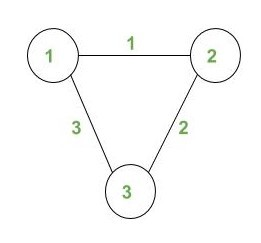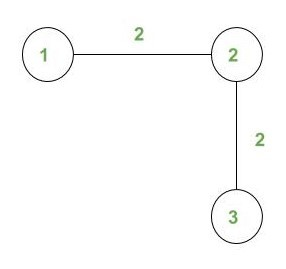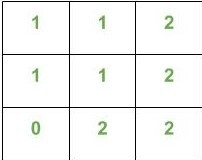# Count the number of walks of length N where cost of each walk is equal to a given number

Given a weighted undirected graph, Length of walks N and Cost X. The task is to count the number of different walks W of length N such that Cost(W) = X.

We define the cost of a walk W, as the maximum over the weights of the edges along the walk.

The nodes are numbered from 1 to n. The graph does not contain any multiple edges or self-loops.

Examples:

Input:.
N = 4, X = 2
Output: 10

Explanation :
A walk W on the graph is a sequence of vertices (with repetitions of vertices and edges allowed) such that every adjacent pair of vertices in the sequence is an edge of the graph.

For X = 2, all possible 10 walks are listed below :

1. 1 -> 2 -> 1 -> 2 -> 3
2. 1 -> 2 -> 3 -> 2 -> 1
3. 1 -> 2 -> 3 -> 2 -> 3
4. 2 -> 1 -> 2 -> 3 -> 2
5. 2 -> 3 -> 2 -> 1 -> 2
6. 2 -> 3 -> 2 -> 3 -> 2
7. 3 -> 2 -> 1 -> 2 -> 1
8. 3 -> 2 -> 1 -> 2 -> 3
9. 3 -> 2 -> 3 -> 2 -> 1
10. 3 -> 2 -> 3 -> 2 -> 3

Input:N = 4, X = 2
Output: 12

## Recommended: Please try your approach on {IDE} first, before moving on to the solution.

1. The idea is to precalculate the no. of walks of length N for each vertex of all possible cost and store them in a 2-D matrix.Let us call this matrix as B.These values can be calculated by running DFS on the given undirected graph.

For example,The given snapshot of matrix B shows the values stored in it. here B(i, j) means no. of walks of length N from vertex i having cost of walk j.

2. We maintain a 1-D array Maxedge in which we keep the cost of walk of length N. We call the same function when the length of walk is less than N and there is some cost X associated with edge(u, v).
We put a base condition for length == N for which we update the array B and return the call.
3. After calculating the matrix B we simply count the total no of walks by adding the no of walks of all the vextex having cost = x.

Ans += B[i][x];
Here i ranges from 1 to n where n is the no of vertices.

Below is the implementation of the above approach

## C++

 `// C++ program to count the number of walks ` `// of length N where cost of each walk is ` `// equal to k ` `#include ` `using` `namespace` `std; ` `int` `G = {0}; ` `int` `Maxedge = {0}; ` `int` `B = {0}; ` `int` `l = 0, n, m; ` ` `  `// Function return total  ` `// walk of length N ` `int` `TotalWalks(``int` `cost) ` `{ ` `     ``int` `ans=0; ` `     `  `    ``// Add values of all  ` `    ``// node with cost X ` `     ``for``(``int` `i=1;i<=n;i++) ` `     ``{ ` `        ``ans+=B[i][cost]; ` `     ``} ` `     `  `     ``return` `ans; ` `} ` ` `  ` `  `// Function to precompute array B ` `// meantioned above ` `void` `DFS(``int` `u, ``int` `v,``int` `len) ` `{ ` `    ``// Base condition ` `    ``if` `(l == len)               ` `    ``{ ` `        ``// Updating the matrix B when  ` `        ``// we get a walk of length N. ` `        ``B[u][ Maxedge[len]]++;  ` `        ``return` `; ` `    ``} ` `    ``for` `(``int` `i = 1; i <= n; i++) ` `    ``{ ` `        ``if` `(G[v][i] !=0) ` `        ``{ ` `            ``// Incrementing the length  ` `            ``// of the walk ` `            ``l++; ` `             `  `            ``// Updating the cost of the walk ` `            ``Maxedge[l] = max(Maxedge[l - 1],  ` `                             ``G[v][i]);  ` `            ``DFS(u, i, len); ` `            ``l--; ` `        ``} ` `    ``} ` `} ` ` `  `// Function to calculate total ` `// number of walks of length N ` `void` `NumberOfWalks(``int` `cost,``int` `len) ` `{ ` `    ``for` `(``int` `i = 1; i <= n; i++) ` `    ``{ ` `        ``// Calling the function DFS ` `        ``DFS(i, i, len);   ` `    ``} ` `     `  `    ``int` `ans = TotalWalks(cost); ` `     `  `    ``// Print the answer ` `    ``cout<< ans << endl; ` `} ` ` `  `// Driver code ` `int` `main() ` `{ ` `    ``int` `Cost = 2; ` `    ``n = 3, m = 3; ` `    ``int` `length = 4; ` `     `  `    ``// Create a graph given in ` `    ``// the above diagram  ` `    ``G = 1; ` `    ``G = 1; ` `    ``G = 2; ` `    ``G = 2; ` `    ``G = 3; ` `    ``G = 3; ` `     `  `    ``NumberOfWalks(Cost, length) ; ` `} `

## Java

 `// Java program to count the number of walks ` `// of length N where cost of each walk is ` `// equal to k ` `import` `java.util.*; ` ` `  `class` `GFG{ ` `     `  `static` `int` `[][]G = ``new` `int``[``250``][``250``]; ` `static` `int` `[]Maxedge = ``new` `int``[``250``]; ` `static` `int` `[][]B = ``new` `int``[``250``][``250``]; ` `static` `int` `l = ``0``, n, m; ` ` `  `// Function return total  ` `// walk of length N ` `static` `int` `TotalWalks(``int` `cost) ` `{ ` `    ``int` `ans = ``0``; ` `     `  `    ``// Add values of all  ` `    ``// node with cost X ` `    ``for``(``int` `i = ``1``; i <= n; i++) ` `    ``{ ` `        ``ans += B[i][cost]; ` `    ``} ` `     `  `    ``return` `ans; ` `} ` ` `  `// Function to precompute array B ` `// meantioned above ` `static` `void` `DFS(``int` `u, ``int` `v, ``int` `len) ` `{ ` `    ``// Base condition ` `    ``if` `(l == len)              ` `    ``{ ` `         `  `        ``// Updating the matrix B when  ` `        ``// we get a walk of length N. ` `        ``B[u][ Maxedge[len]]++;  ` `        ``return``; ` `    ``} ` `     `  `    ``for` `(``int` `i = ``1``; i <= n; i++) ` `    ``{ ` `        ``if` `(G[v][i] !=``0``) ` `        ``{ ` `             `  `            ``// Incrementing the length  ` `            ``// of the walk ` `            ``l++; ` `             `  `            ``// Updating the cost of the walk ` `            ``Maxedge[l] = Math.max(Maxedge[l - ``1``],  ` `                                        ``G[v][i]);  ` `            ``DFS(u, i, len); ` `            ``l--; ` `        ``} ` `    ``} ` `} ` ` `  `// Function to calculate total ` `// number of walks of length N ` `static` `void` `NumberOfWalks(``int` `cost,``int` `len) ` `{ ` `    ``for``(``int` `i = ``1``; i <= n; i++) ` `    ``{ ` ` `  `       ``// Calling the function DFS ` `       ``DFS(i, i, len);  ` `    ``} ` `     `  `    ``int` `ans = TotalWalks(cost); ` `     `  `    ``// Print the answer ` `    ``System.out.print(ans + ``"\n"``); ` `} ` ` `  `// Driver code ` `public` `static` `void` `main(String[] args) ` `{ ` `    ``int` `Cost = ``2``; ` `    ``n = ``3``; m = ``3``; ` `    ``int` `length = ``4``; ` `     `  `    ``// Create a graph given in ` `    ``// the above diagram  ` `    ``G[``1``][``2``] = ``1``; ` `    ``G[``2``][``1``] = ``1``; ` `    ``G[``2``][``3``] = ``2``; ` `    ``G[``3``][``2``] = ``2``; ` `    ``G[``1``][``3``] = ``3``; ` `    ``G[``3``][``1``] = ``3``; ` `     `  `    ``NumberOfWalks(Cost, length); ` `} ` `} ` ` `  `// This code is contributed by 29AjayKumar `

## C#

 `// C# program to count the number of walks ` `// of length N where cost of each walk is ` `// equal to k ` `using` `System; ` ` `  `class` `GFG{ ` `     `  `static` `int` `[,]G = ``new` `int``[250, 250]; ` `static` `int` `[]Maxedge = ``new` `int``; ` `static` `int` `[,]B = ``new` `int``[250, 250]; ` `static` `int` `l = 0, n; ` ` `  `// Function return total  ` `// walk of length N ` `static` `int` `TotalWalks(``int` `cost) ` `{ ` `    ``int` `ans = 0; ` `     `  `    ``// Add values of all  ` `    ``// node with cost X ` `    ``for``(``int` `i = 1; i <= n; i++) ` `    ``{ ` `       ``ans += B[i, cost]; ` `    ``} ` `    ``return` `ans; ` `} ` ` `  `// Function to precompute array B ` `// meantioned above ` `static` `void` `DFS(``int` `u, ``int` `v, ``int` `len) ` `{ ` ` `  `    ``// Base condition ` `    ``if` `(l == len)              ` `    ``{ ` `         `  `        ``// Updating the matrix B when  ` `        ``// we get a walk of length N. ` `        ``B[u, Maxedge[len]]++;  ` `        ``return``; ` `    ``} ` `     `  `    ``for``(``int` `i = 1; i <= n; i++) ` `    ``{ ` `       ``if` `(G[v, i] != 0) ` `       ``{ ` `            `  `           ``// Incrementing the length  ` `           ``// of the walk ` `           ``l++; ` `            `  `           ``// Updating the cost of the walk ` `           ``Maxedge[l] = Math.Max(Maxedge[l - 1],  ` `                                       ``G[v, i]);  ` `           ``DFS(u, i, len); ` `           ``l--; ` `       ``} ` `    ``} ` `} ` ` `  `// Function to calculate total ` `// number of walks of length N ` `static` `void` `NumberOfWalks(``int` `cost, ``int` `len) ` `{ ` `    ``for``(``int` `i = 1; i <= n; i++) ` `    ``{ ` `        `  `       ``// Calling the function DFS ` `       ``DFS(i, i, len);  ` `    ``} ` `     `  `    ``int` `ans = TotalWalks(cost); ` `     `  `    ``// Print the answer ` `    ``Console.Write(ans + ``"\n"``); ` `} ` ` `  `// Driver code ` `public` `static` `void` `Main(String[] args) ` `{ ` `    ``int` `Cost = 2; ` `    ``n = 3; ` `    ``int` `length = 4; ` `     `  `    ``// Create a graph given in ` `    ``// the above diagram  ` `    ``G[1, 2] = 1; ` `    ``G[2, 1] = 1; ` `    ``G[2, 3] = 2; ` `    ``G[3, 2] = 2; ` `    ``G[1, 3] = 3; ` `    ``G[3, 1] = 3; ` `     `  `    ``NumberOfWalks(Cost, length); ` `} ` `} ` ` `  `// This code is contributed by gauravrajput1 `

Output:

```10
```

Attention reader! Don’t stop learning now. Get hold of all the important DSA concepts with the DSA Self Paced Course at a student-friendly price and become industry ready.

My Personal Notes arrow_drop_upCheck out this Author's contributed articles.

If you like GeeksforGeeks and would like to contribute, you can also write an article using contribute.geeksforgeeks.org or mail your article to contribute@geeksforgeeks.org. See your article appearing on the GeeksforGeeks main page and help other Geeks.

Please Improve this article if you find anything incorrect by clicking on the "Improve Article" button below.

Improved By : 29AjayKumar, GauravRajput1

Article Tags :
Practice Tags :

Be the First to upvote.

Please write to us at contribute@geeksforgeeks.org to report any issue with the above content.